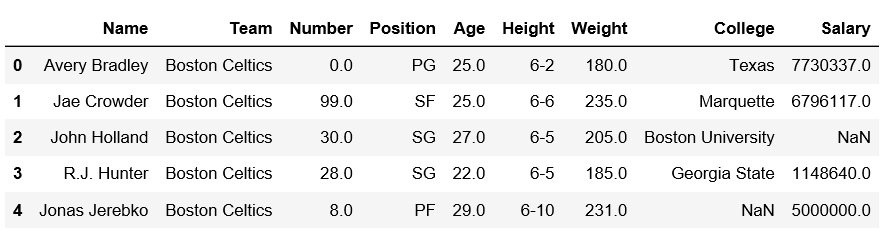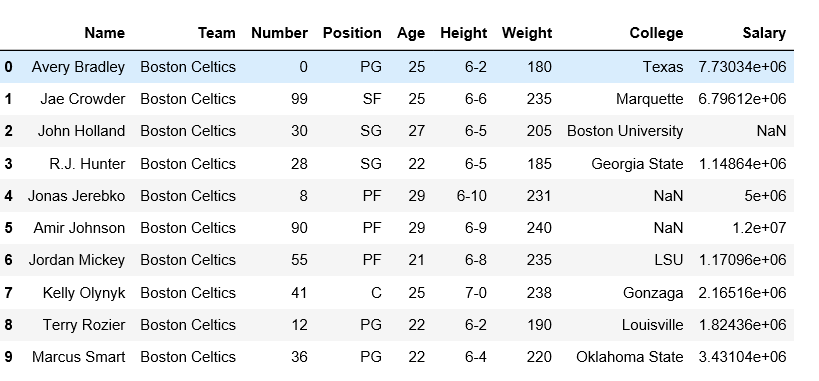GeeksforGeeks App
Open AppBrowser
Continue

# Reshape a pandas DataFrame using stack,unstack and melt method

Pandas use various methods to reshape the dataframe and series. Let’s see about the some of that reshaping method.

Let’s import a dataframe first.

 `# import pandas module``import` `pandas as pd`` ` `# making dataframe``df ``=` `pd.read_csv(``"https://media.geeksforgeeks.org/wp-content/uploads/nba.csv"``)`` ` `# it was print the first 5-rows``print``(df.head()) `

Output:Using stack() method:

Stack method works with the MultiIndex objects in DataFrame, it returning a DataFrame with an index with a new inner-most level of row labels. It changes the wide table to a long table.

 `# import pandas module``import` `pandas as pd`` ` `# making dataframe``df ``=` `pd.read_csv(``"nba.csv"``)`` ` `# reshape the dataframe using stack() method``df_stacked ``=` `df.stack()`` ` `print``(df_stacked.head(``26``))`

Output:Using unstack() method:
`unstack `is similar to stack method, It also works with multi-index objects in dataframe, producing a reshaped DataFrame with a new inner-most level of column labels.

 `# import pandas module``import` `pandas as pd`` ` `# making dataframe``df ``=` `pd.read_csv(``"nba.csv"``)`` ` `# unstack() method``df_unstacked ``=` `df_stacked.unstack()``print``(df_unstacked.head(``10``))`Using `melt()` method:
Melt in pandas reshape dataframe from wide format to long format. It uses the “id_vars[‘col_names’]” for melt the dataframe by column names.

 `# import pandas module``import` `pandas as pd`` ` `# making dataframe``df ``=` `pd.read_csv(``"nba.csv"``)`` ` `# it takes two columns "Name" and "Team"``df_melt ``=` `df.melt(id_vars ``=``[``'Name'``, ``'Team'``]) ``print``(df_melt.head(``10``))`

Output:My Personal Notes arrow_drop_up